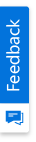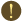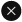We use cookies to give you the best experience on our website. If you continue to browse, then you agree to our privacy policy and cookie policy.Unfortunately, activation email could not send to your email. Please try again.# Creating dynamic application driven by excelling formulas using CircularGauge

Platform: WPF |
Control: SfCircularGauge |
Published Date: July 18, 2014 |
Last Revised Date: June 26, 2019 |
Product Version: 12.1.0.43
Tags:

You can create dynamic application driven by excel formulas using Circular Gauges and update the Gauge pointers based on the cell values of an Excel sheet. To achieve this requirement, you need to consider the following three areas,

• UI Design and Pointer Value Update
• Connect with Excel
• Get and Set the Cell Values

## UI Design and Pointer Value Update

The project has been created using SfCircularGauge and XlsIO controls. Refer the following namespaces.

C#

```using Syncfusion.UI.Xaml.Gauges;
using Syncfusion.XlsIO;
```

Note:

Implementation and APIs are similar in both WinRT and WPF Platforms. You can refer the mentioned UG link for WinRT platform.Figure 1: Car Dashboard

The above sample contains five Circular Gauges and you can update its value for every 10 seconds.  You can use DispatcherTimer to update pointer values in its ticks.

C#

``` private DispatcherTimer timer;
//////

timer = new DispatcherTimer();
timer.Interval = new TimeSpan(0, 0, 0, 10);
timer.Tick += timer_Tick;

//////

void timer_Tick(object sender, object e)
{
//code to update pointer values
}
```

To change the values of the cells in the Excel sheet, you can use Numeric Up Down control.Figure 2 : Numeric Up Down control to change Cell values

## Connect with Excel

To connect with the Excel sheet (where you have the values for Gauge pointers), you can maintain a class with some necessary properties in it.

C#

``` public  class ExcelConnect : FrameworkElement
{
public ExcelRange Range;
public string ExcelFile
public string SheetName;

if (ExcelFile != string.Empty && SheetName != string.Empty)
{
m_workBook = m_eng.Excel.Workbooks.Open(ExcelFile, ExcelOpenType.Automatic);
}
}
```

You can easily connect and access the required Excel Sheet by maintaining the following class.

Xaml

``` <local:ExcelConnect x:Name="excelConnect1" ExcelFile="..\..\projection.xls" SheetName="Sheet1"/>
```

## Get and Set the Cell Values

Step 1: To get the cell Value from the excel sheet

The following code example explains how to retrieve the particular cell value from the Excel sheet.

C#

```public override bool TryGetMember(
GetMemberBinder binder, out object result)
{
result = Connection.GetRangeValuesFromExcel(range);
}

public object GetRangeValuesFromExcel(string range)
{
return GetFromExcel(range);
}

public string GetFromExcel(string range)
{
return Sheet[range].CalculatedValue;
}
```

Step 2: To set the cell values for the pointers

You can set the cell values for each Gauge pointer in the timer tick event to update for every ten seconds.

C#

``` void timer_Tick(object sender, object e)
{
Speed = Double.Parse((this.excelConnect1.Sheet as IWorksheet)["B4"].CalculatedValue);            RPM = Double.Parse((this.excelConnect1.Sheet as IWorksheet)["C4"].CalculatedValue);            Temperature = Double.Parse((this.excelConnect1.Sheet as IWorksheet)["D4"].CalculatedValue);            Fuel = Double.Parse((this.excelConnect1.Sheet as IWorksheet)["E4"].CalculatedValue);            Torque = Double.Parse((this.excelConnect1.Sheet as IWorksheet)["F4"].CalculatedValue);
}
```

Step 3: To change the cell value through the Numeric Up Down control

You can bind the cell value with the numeric up down control value as mentioned in the following code example.

Xaml

```<syncfusion:UpDown Height="26" HorizontalAlignment="Left" Name="upDown1" VerticalAlignment="Top" Width="90" Value="{Binding ElementName=excelConnect1, Path=Range.B4, Mode=TwoWay}" />
```

Step 4: To set or change the cell Value in the Excel sheet.

You can change/set (to write) the value on the Excel sheet as mentioned in the following code example.

C#

``` public void WriteToExcel(string range, object cellValue)
{
if (cellRange.Cells.Length == 1)
{
cellRange.Value2 = cellValue;
}
}
```

2X faster development

The ultimate WPF UI toolkit to boost your development speed.You are using an outdated version of Internet Explorer that may not display all features of this and other websites. Upgrade to Internet Explorer 8 or newer for a better experience.